# Dickman-de Bruijn function

## History

The function was first introduced by Dickman with a heuristic argument relating it to smoothness. de Bruijn explored many properties of this function, and Ramaswami gave a formal proof of its relation to the size of the largest prime divisor.

## Definition

This function, called Dickman's function or the Dickman-de Bruijn function, is defined as the function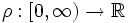satisfying the delay differential equation: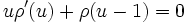subject to the initial condition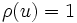for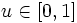. The function satisfies the following properties:

•for.
•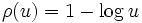for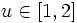.
•is (strictly) decreasing for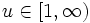, i.e.,for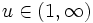.
•is once differentiable on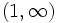. More generally, \$\rho[/itex] istimes differentiable everywhere except at the points.
•is infinitely differentiable except at integers.
•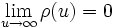.

It turns out that the density of numbers with no prime divisor greater than theroot is given by. Formally, consider, for any, the fraction of natural numbers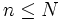such that all prime divisors ofare at most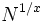. Then, as, this fraction tends to. Thus, this function is crucial to understand the behavior of the largest prime divisor function and it is important in obtaining smoothness bounds.